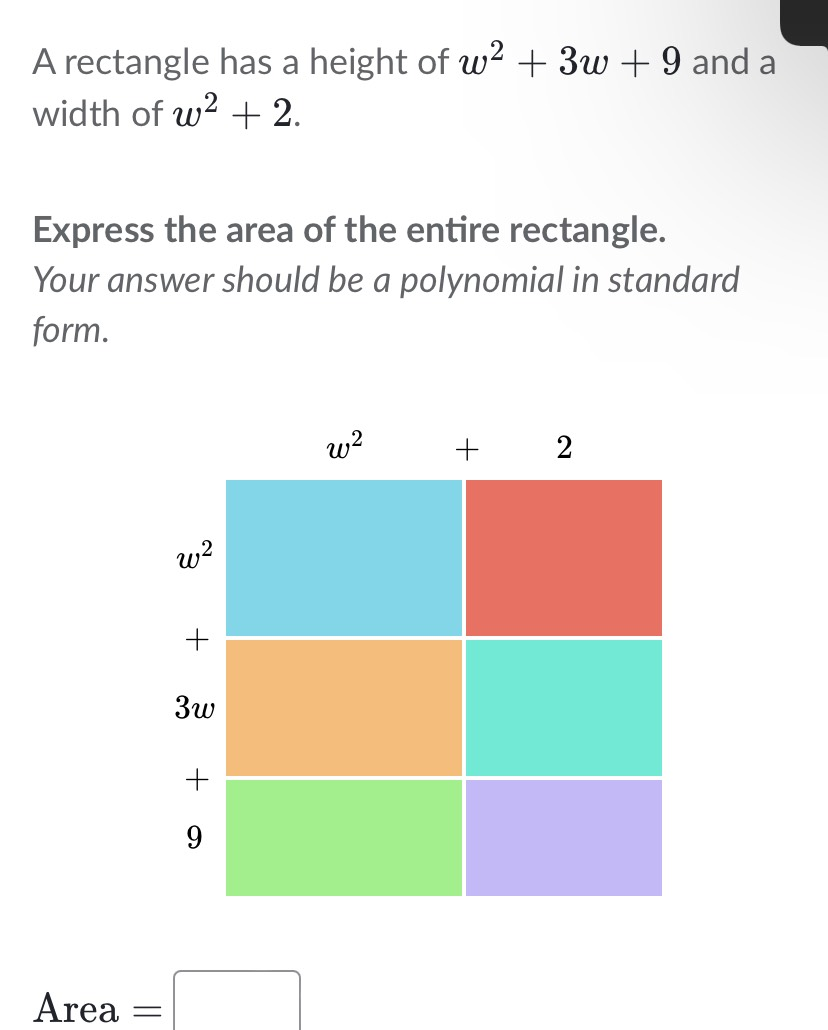### ¿Todavía tienes preguntas de matemáticas?

Pregunte a nuestros tutores expertos
Algebra
PreguntaA rectangle has a height of $$w ^ { 2 } + 3 w + 9$$ and a width of $$w ^ { 2 } + 2$$

Express the area of the entire rectangle. Your answer should be a polynomial in standard form.

$$w^4+ 3w^3+ 11w^2+ 6w+ 18$$